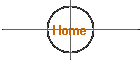## Why are orbits elliptical?  Why isn't the Sun at the center of the orbits?  Is there a mathematical or gravitational answer or is it just random placement after formation?

These are great questions about elliptical orbits.  To answer the primary question briefly -  It's  because of physics that the orbits are nearly always elliptical.  Of course, nothing precludes a perfectly circular orbit other than the fact that it would be very unusual for any orbit to be PERFECTLY circular.  The situation where the Sun, for instance, would be exactly in the center of a perfectly circular planetary orbit couldn't happen in our Solar System since there are other planets that would gravitationally affect the orbit and cause it to be immediately non-circular by pulling the object to one side or the other.  Any other stable orbit type than a perfectly circular one turns out to be an elliptical orbit.

"An ellipse is defined as a figure drawn around two points called the foci in such a way that the distance from one focus to any point on the ellipse and then back to the other focus equals a constant.  This makes it very easy to draw ellipses with two thumbtacks and a loop of string (both ends tied together).  Press the thumbtacks into a board, loop the string about them, and place a pencil in the loop.  If you keep the string taught as you move the pencil, it will trace out an ellipse." - from Michael A. Seeds, "Horizons - Exploring the Universe," Wadsworth Publishing Company.  A great freshman non-science major astronomy textbook.

You can think about it in this way...  Because of geometry and because of Newton's law of universal gravitation (* see notes below), there are only four different paths that an object passing close to the Sun might take.  The first would be a hyperbolic orbit - for example, the object comes in from space towards the Sun with so much energy that it swings around the Sun, but still with enough energy to head back out into space on a different course...  An path that would look kind of like a stretched out letter "U".  Secondly, the object could come in from space without enough energy to escape from the Sun's gravity and would thus be captured in an elliptical orbit with the eccentricity of the orbit dependent upon the object's initial energy.  Thirdly, the object might have PRECISELY the amount of energy to be captured in a perfectly circular orbit.  And finally fourth, the object might not have enough energy to establish any sort of orbit and it would spiral into the surface of the Sun and burn up.

These are the only theoretical choices:  hyperbolic, elliptical, circular, and spiral (impact).

So what really happens in our solar system?  It is believed that there are some comets from the distant fringe of our Solar System, from the Oort cloud, that fall into the inner Solar System, swing around the Sun and then head back out into space on hyperbolic orbits, never to return for another pass around the Sun...I say 'believed' because for an orbit to be defined as hyperbolic, the comet can *never* return.  Not even in millions and millions of years.  If it does, then the orbit is classified as elliptical with extremely high eccentricity.  (Please reference any  geometry textbook).  Most solar system objects that we are familiar with like the planets, asteroids, even the particles in the rings of Saturn are all in elliptical orbits.  Most of these orbits are very nearly circular, but once again, to be considered "circular" they have to be perfectly circular.  OK, that horse is beaten to death. :-)

While the familiar objects are in elliptical orbits, there are a lot of objects that fall into the Sun on a spiral path.  Comets die this way all of the time.  You can do an online search for the SOHO space observatory to find pictures it has captured of this actually occurring.  To my knowledge, there is nothing in a truly circular orbit around the Sun.

Back to the question of "Why?"  I'd like to offer you a reference that you can find at your local library.  Please look up the college freshman Physics textbook by Robert Resnick and David Halliday, published by John Wiley & Sons.  Not only is there a complete explanation of Kepler's First Law, but also of gravitation, and Kepler's other two laws, very much related to your question.

As a side note to all of this, scientists call an equation a "Law" when they discover something that they see as fundamental to the way entire universe works and that has never been contradicted by other empirical evidence that can't be explained.  The whole strength behind Kepler's First Law was that it allowed for the future positions of the planets to be predicted precisely far into the future, something that had never been possible before with the previously proposed complicated schemes.  The elliptical orbit idea was so simple while neatly explaining and predicting planetary motion extremely well that it was seen as a fundamental revolution in scientific thinking.  His laws explained the facts of how scientists knew the universe behaved.  To quest to answer 'why' gravity works in the way it does is one of the areas of theoretical physics and cosmological research today.

* Note 1

Universal Law of Gravitation:
F = G * (m1 * m2) / r2

F = gravitational force
G = Gravitational constant       (6.67x10-11 N-m2/kg2)
m1 = mass of first object
m2 = mass of second object
r = distance between m1 and m2

* Note 2
Equation of an ellipse:
If 2a = length of major axis and if
2b = length of minor axis

then the equation of an ellipse in rectangular coordinates (X, Y) is:
(X-Xo)2 / a2 + (Y-Yo)2 / b2 = 1

* Note 3
Other suggested references:
Geometry textbook
Trigonometry textbook

11/2011
 10/01/2017